# Color temperature (Kelvin) to RGB conversion: Algorithms and Sample

As far as I know, it is unlikely to find a reliable formula for converting from the color temperature to RGB. Although, there are many algorithms to achieve similar functionality, however, are mostly converted from the color temperature to XYZ color space. After that you can re-needed space in the conversion from XYZ to RGB color space. One implementation of this algorithm you can find here.

Unfortunately, this approach is not a mathematical formula in the true sense, but a way to achieve the look-up table landscaping. In some cases, this is a reliable implementation. However, when it comes to XYZ-> RGB conversion time, for simple adjustment of the color temperature in real time, in this way too slow.

So, I set the color temperature to achieve the RGB conversion algorithm. The conversion algorithm is quite good. The following are some of my ideas.

## Use some of the limitations of this algorithm:

1. My algorithm is a highly accurate estimate. However, in terms of scientific accuracy is not enough. This algorithm is mainly used in the treatment of some photos, so do not try to use this algorithm in astronomy and medical image processing.
2. Since the algorithm is relatively simple, the image processing algorithm suitable size, real time has rapidly enough. However, in order to obtain better real-time performance, you must implement the programming language you are using this algorithm and then do some optimization.
3. This algorithm applies only to convert 1000K to 40000K color temperature of. For photography for such a large spectral range is sufficient. When using the color temperature exceeds this range, the accuracy will decrease.

## Algorithm: Sample output

FIG algorithm output is the image of 1000K to 40000K.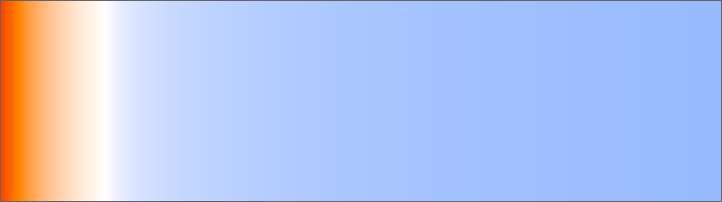FIG lower color temperature range of interest in photography: from 1500K to 15000K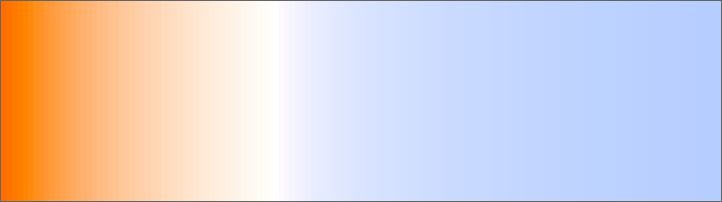Algorithm thinking

My first step is to work from the Charity's original blackbody values to achieve a reliable formula for reverse engineering method.

Charity's original blackbody values of the data represented graphically as: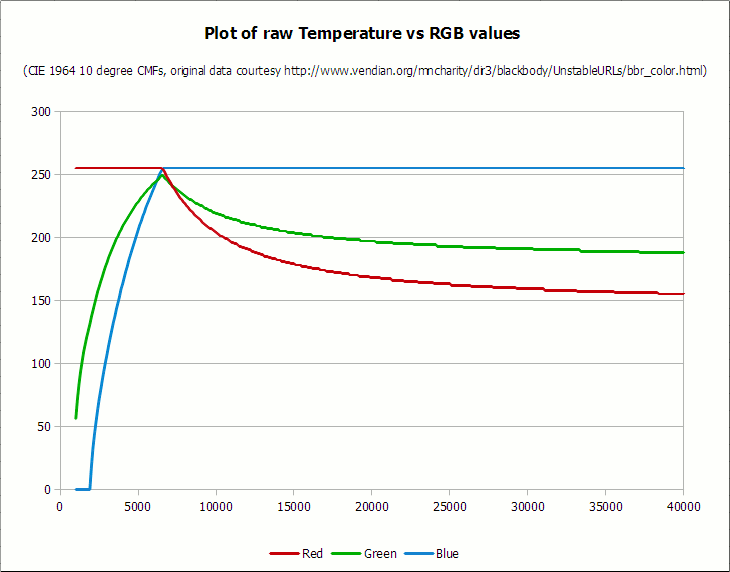1. 红色分量在6600K以下的时候总保持255
2. 蓝色分量在2000K的时候总保持0
3. 蓝色问了在6500K以上总保持255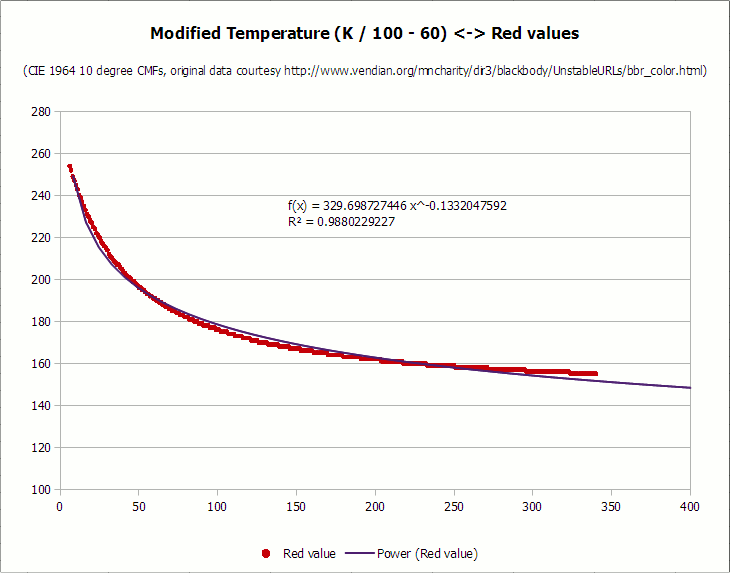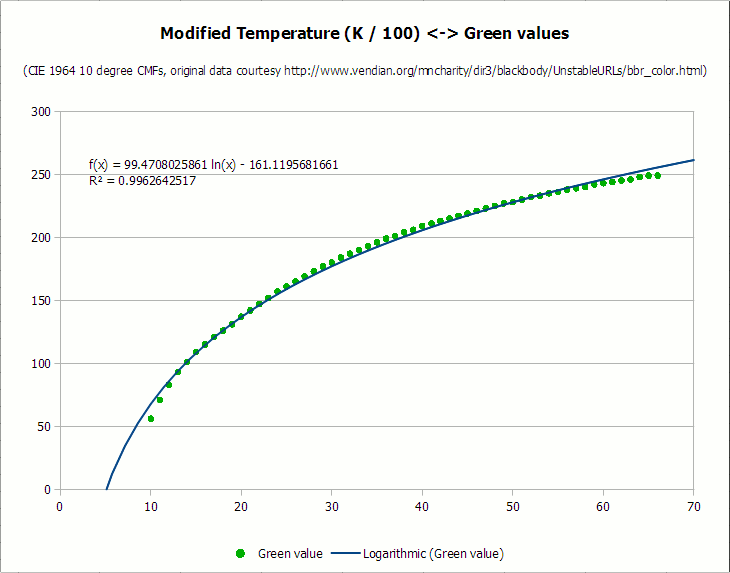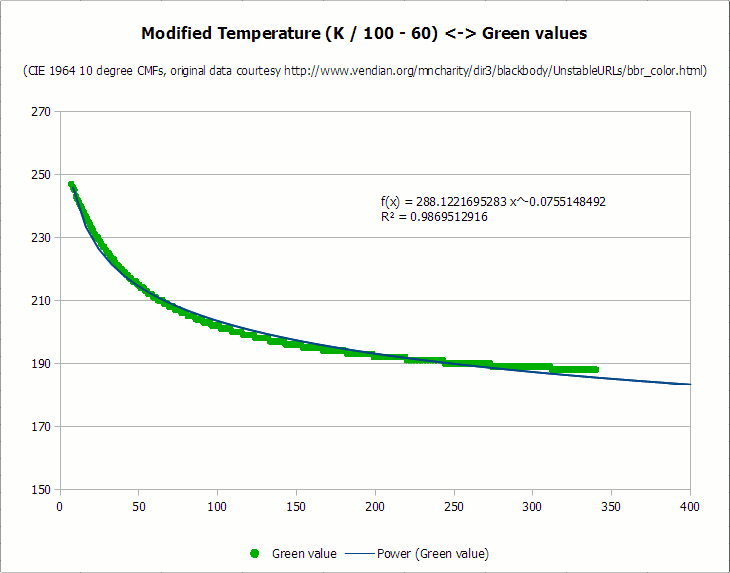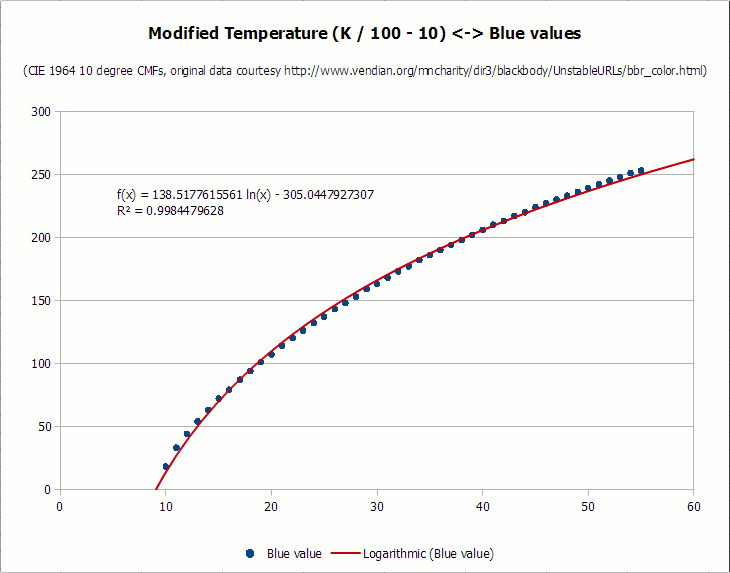``````输入：1000K到40000K的色温；色温和颜色分量的变量必须是双精度

Set Temperature = Temperature \ 100

Calculate Red:

If Temperature <= 66 Then

Red = 255

Else

Red = Temperature - 60

Red = 329.698727446 * (Red ^ -0.1332047592)

If Red < 0 Then Red = 0

If Red > 255 Then Red = 255

End If

Calculate Green:

If Temperature <= 66 Then

Green = Temperature

Green = 99.4708025861 * Ln(Green) - 161.1195681661

If Green < 0 Then Green = 0

If Green > 255 Then Green = 255

Else

Green = Temperature - 60

Green = 288.1221695283 * (Green ^ -0.0755148492)

If Green < 0 Then Green = 0

If Green > 255 Then Green = 255

End If

Calculate Blue:

If Temperature >= 66 Then

Blue = 255

Else

If Temperature <= 19 Then

Blue = 0

Else

Blue = Temperature - 10

Blue = 138.5177312231 * Ln(Blue) - 305.0447927307

If Blue < 0 Then Blue = 0

If Blue > 255 Then Blue = 255

End If

End If``````

``````Static tmpCalc As Double

'Temperature must fall between 1000 and 40000 degrees

If tmpKelvin < 1000 Then tmpKelvin = 1000

If tmpKelvin > 40000 Then tmpKelvin = 40000

'All calculations require tmpKelvin \ 100, so only do the conversion once

tmpKelvin = tmpKelvin \ 100

'Calculate each color in turn

'First: red

If tmpKelvin <= 66 Then

r = 255

Else

'Note: the R-squared value for this approximation is .988

tmpCalc = tmpKelvin - 60

tmpCalc = 329.698727446 * (tmpCalc ^ -0.1332047592)

r = tmpCalc

If r < 0 Then r = 0

If r > 255 Then r = 255

End If

'Second: green

If tmpKelvin <= 66 Then

'Note: the R-squared value for this approximation is .996

tmpCalc = tmpKelvin

tmpCalc = 99.4708025861 * Log(tmpCalc) - 161.1195681661

g = tmpCalc

If g < 0 Then g = 0

If g > 255 Then g = 255

Else

'Note: the R-squared value for this approximation is .987

tmpCalc = tmpKelvin - 60

tmpCalc = 288.1221695283 * (tmpCalc ^ -0.0755148492)

g = tmpCalc

If g < 0 Then g = 0

If g > 255 Then g = 255

End If

'Third: blue

If tmpKelvin >= 66 Then

b = 255

ElseIf tmpKelvin <= 19 Then

b = 0

Else

'Note: the R-squared value for this approximation is .998

tmpCalc = tmpKelvin - 10

tmpCalc = 138.5177312231 * Log(tmpCalc) - 305.0447927307

b = tmpCalc

If b < 0 Then b = 0

If b > 255 Then b = 255

End If

End Sub``````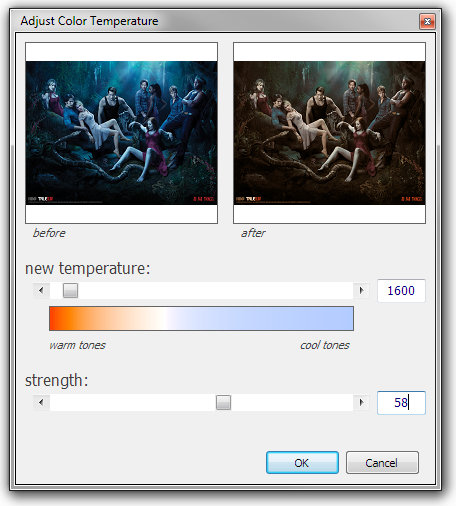### Guess you like

Origin blog.csdn.net/lz0499/article/details/104088393
Recommended
Ranking
Daily